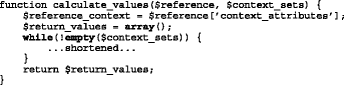# Table 3 Design pattern on the conceptual level (example)

Component term Context similarity
Subset Absolute congruence
Description This component uses an absolute congruence metric as similarity measure to identify context similarity.
Problem description Reputation data is always bound to the specific context in which it was created. Ratings that were generated in one application area might not be automatically applicable in another application area which can result in the value imbalance problem.
Solution description Apply similarity measurement between context c i (reference context) and context c j of referrals in the referral set to deliver a weight-factor for each item of the referral set using the following formula: $$w(c_{1},c_{2}):= \frac {k(c_{i}) \cap k(c_{j})}{k(c_{i}) \cup k(c_{j})}$$ k(c i ) denotes the total number of keywords describing context c i .
Applicability Set of nominal context attributes.
Code example (php)Implementation Context similarity-based weighting service (absolute congruence)
Literature • Mohammad Gias Uddin, Mohammad Zulkernine, and Sheikh Iqbal Ahamed. 2008. CAT: a context-aware trust model for open and dynamic systems. In Proceedings of the 2008 ACM symposium on Applied computing (SAC ’08). ACM, New York, NY, USA, 2024–2029.
Tags weighting, context, similarity, congruence